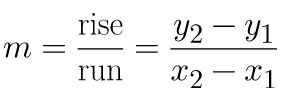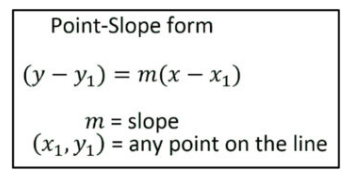Search

# What is the Line of Best Fit and What is its Significance?

Did you know that the Line of Best Fit is the sometimes called as the Trend Line or the Line of Regression?

Yes!!

In fact, when we represent data in the form of a scatter plot, we are able to see how one variable affects the other. And when data follows a similar pattern, this relationship is called correlation.

We represent this correlation by using trend lines or best fit lines that help us to approximate a set of data points.

First we must construct a scatter plot from the given data and try to understand correlation.Types of Correlation

Next, we sketch the line that appears to most closely follow the correlation. We have to construct a line that best represents the trend. Most likely, we are looking at the median values, which is why the trend line is sometimes called the median fit line.Then we find 2 points that lie on the regression line and calculate the slope, m.Finally, we use Point Slope Form to write the linear equation that represents the line of best fit.In the above scatter plot, the green line is the line of best fit.

Now, in actual scenario, not every data point will be on our line of best fit. Consequently, there will be points above and below our line. This error in our prediction is called a residual and it is the vertical distance between a data point and the regression line. The better the line fits the data, the smaller the residuals (on average).In other words, some of the actual values will be larger than their predicted value (they will fall above the line), and some of the actual values will be less than their predicted values (they'll fall below the line).We have to think of some combination of all the residuals and try to minimize it.

Some of the errors will be positive and some will be negative. So , If we add up all of the errors, the sum will be zero.

So, in order to measure the overall error, we square the errors and find a line that minimizes this sum of the squared errors. When we square an error , the errors will become large and the sum of the squared errors will be a bigger proportion of the Sum.

Observed /Actual Y - Predicted Y = Error

Y - Y' = Y-Y'

Then we take the square of the error = (Y-Y')^2

Sum of all the squared error = Mean Squared Error.This method, the method of least squares, finds values of the intercept(b) and slope coefficient(m) that minimizes the sum of the squared errors.

This method is very valuable because it takes into account the significant outliers - the points that are far away from the model .

Hence when we use this method to calculate our line of best fit equation, Our line of Best Fit will indeed be the Best !

Thanks for stopping by !